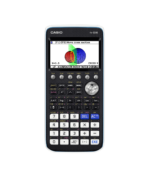Plot and Analyse Functions Archives - Casio Calculators

# Plot and Analyse Functions

Displaying Resources

FilterHow to Video
fx-CG50 GCSE IB A-Level
Quick Skill: Calculations Using Coordinate Values

How to extract coordinate values from points of interest in Graph to perform ope...How to Video
fx-CG50 A-Level GCSE IB
Quick Skill: Graphing – Storing and Recalling Functions

How to use Graph Memory to store functions and settings for later recall. You wi...How to Video
fx-CG50 A-Level GCSE IB
Quick Skill: Table – Extracting Values

How to extract either a single column or a complete set of table values. You wil...How to Video
fx-CG50 GCSE IB A-Level
Quick Skill: Graphing – Drawing a Graph and Table

How to draw the graph of a function and create a table of values from the graph....How to Video
fx-CG50 A-Level GCSE IB
Quick Skill: Graphing – Drawing 2 Graphs

How to draw adjacent graphs for comparing functions. You will need to know how t...How to Video
fx-CG50 A-Level GCSE IB
Quick Skill: Graphs – Quick Scale Settings

How to use Zoom to automatically fill the graph with the sketched function, zoom...How to Video
fx-CG50 A-Level IB
Quick Skill: Graphing – Inverse Trigonometric Functions

How to graph the inverse trigonometric functions. Restricting the domain ensures...How to Video
fx-CG50 IB A-Level
Quick Skill: Graphing – Restricting the Domain of a Function

How to restrict the domain of a function when graphing. You will need to know ho...How to Video
fx-CG50 A-Level IB
Quick Skill: Calculations Using Function Notation

How to use function notation to perform operations in the Run-Matrix app. You wi...How to Video
fx-CG50 A-Level IB
Modulus Function – Graphs and Equations

How to plot the graph of a modulus function. Solve modulus equations both graphi...How to Video
fx-CG50 A-Level GCSE IB
Graphs – Key Points and Intersections

How to find the coordinates of key points such as intercepts and stationary poin...How to Video
fx-CG50 GCSE IB A-Level
Graphs – Getting Started

How to plot the graph of a function, adjust the axes of a graph, move around the...How to Video
fx-CG50 A-Level IB
Graphing – Types of Function

Plot graphs of parametric functions and where x = constant. Plot inequalities an...Teach Leaflet
fx-CG50 A-Level GCSE IB
Intersection of a Line and Curve

This activity asks learners to divide a set of 10 cards into four subsets accord...Teach Leaflet
fx-CG50 A-Level GCSE IB